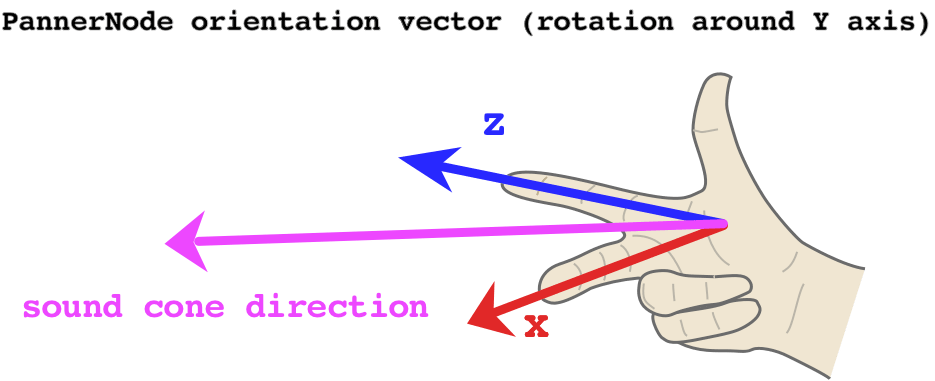# PannerNode.coneOuterAngle

The `coneOuterAngle` property of the `PannerNode` interface is a double value describing the angle, in degrees, of a cone outside of which the volume will be reduced by a constant value, defined by the `coneOuterGain` property.

The `coneOuterAngle` property's default value is `0`.

## Syntax

``````var audioCtx = new AudioContext();
var panner = audioCtx.createPanner();
panner.coneOuterAngle = 0;``````

A double.

## Example

In this example, we'll demonstrate how changing the orientation parameters of a `PannerNode` in combination with `coneInnerAngle` and `coneOuterAngle` affects volume. To help us visualise how the orientation vector affects, we can use the Right-hand rule:First, let's start by writing a utility function to figure out our orientation vector. The X and Z components are always at a 90° to each other, so we can use the sine and cosine functions, which are offset by the same amount in radians. However, normally this would mean the `PannerNode` points to the left of the listener at 0° rotation – since `x = cos(0) = 1` and `z = sin(0) = 0`. It's more useful to offset the angle by -90°, which means the `PannerNode` will point directly at the listener at 0° rotation.

``````// this utility converts amount of rotation around the Y axis
// (i.e. rotation in the 'horizontal plane') to an orientation vector
const yRotationToVector = degrees => {
// convert degrees to radians and offset the angle so 0 points towards the listener
const radians = (degrees - 90) * (Math.PI / 180);
// using cosine and sine here ensures the output values are always normalized
// i.e. they range between -1 and 1
const x = Math.cos(radians);
const z = Math.sin(radians);

// we hard-code the Y component to 0, as Y is the axis of rotation
return [x, 0, z];
};
``````

Now we can create our `AudioContext`, an oscillator and a `PannerNode`:

``````const context = new AudioContext();

const osc = new OscillatorNode(context);
osc.type = 'sawtooth';

const panner = new PannerNode(context);
panner.panningModel = 'HRTF';``````

Next, we set up the cone of our spatialised sound, determining the area in which it can be heard:

``````// this value determines the size of the area in which the sound volume is constant
// if coneInnerAngle == 30, it means that when the sound is rotated
// by at most 15 (30/2) degrees either direction, the volume won't change
panner.coneInnerAngle = 30;
// this value determines the size of the area in which the sound volume decreases gradually
// if coneOuterAngle == 45 and coneInnerAngle == 30, it means that when the sound is rotated
// by between 15 (30/2) and 22.5 (45/2) degrees either direction,
// the volume will decrease gradually
panner.coneOuterAngle = 45;
// this value determines the volume of the sound outside of both inner and outer cone
// setting it to 0 means there is no sound, so we can clearly hear when we leave the cone
// 0 is also the default
panner.coneOuterGain = 0;
// increase the Z position to ensure the cone has an effect
// (otherwise the sound is located at the same position as the listener)
panner.positionZ.setValueAtTime(1, context.currentTime);``````

Having set up the `PannerNode`, we can now schedule some updates to its Y-axis rotation:

``````// calculate the vector for no rotation
// this means the sound will play at full volume
const [x1, y1, z1] = yRotationToVector(0);
// schedule the no-rotation vector immediately
panner.orientationX.setValueAtTime(x1, context.currentTime);
panner.orientationY.setValueAtTime(y1, context.currentTime);
panner.orientationZ.setValueAtTime(z1, context.currentTime);

// calculate the vector for -22.4 degrees
// since our coneOuterAngle is 45, this will just about make the sound audible
// if we set it to +/-22.5, the sound volume will be 0, as the threshold is exclusive
const [x2, y2, z2] = yRotationToVector(-22.4);
panner.orientationX.setValueAtTime(x2, context.currentTime + 2);
panner.orientationY.setValueAtTime(y2, context.currentTime + 2);
panner.orientationZ.setValueAtTime(z2, context.currentTime + 2);``````

Finally, let's connect all our nodes and start the oscillator!

``````osc.connect(panner)
.connect(context.destination);

osc.start(0);
``````

## Specifications

Specification Status Comment
Web Audio API
The definition of 'coneOuterAngle' in that specification.
Working Draft

## Browser compatibility

BCD tables only load in the browser